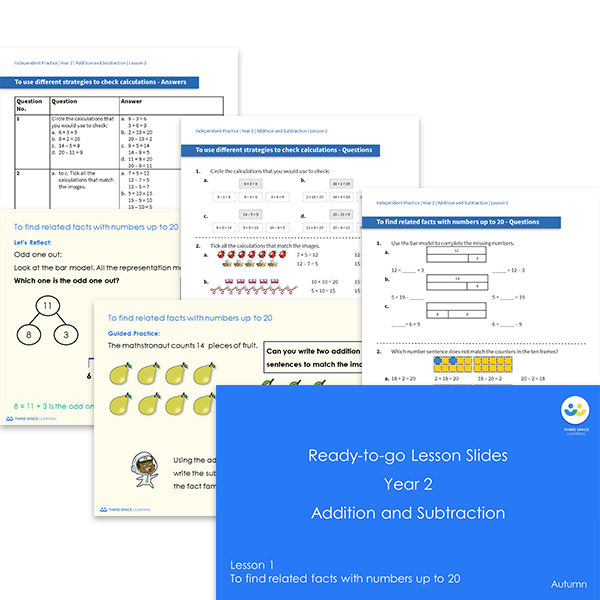# Ready-to-go lessons Year 2 Addition and Subtraction (Autumn Block 2) Slides and Worksheets

Addition and subtraction ready-to-go lessons for Year 2 includes 16 PowerPoints with matching worksheets ready for you to use with a class. These resources follow a mastery approach to mathematics teaching, similar to schemes such as White Rose Maths and Mathematics Mastery. The PowerPoints are fully editable with detailed notes for every teaching slide. Each associated worksheet is divided into three parts with a range of activities to complete across the block.

This Year 2 Addition and Subtraction pack contains 16 fully editable PowerPoints and 16 worksheets.

The slides and worksheets cover:

• Finding related facts (up to 20) and using known facts
• Using different strategies to check calculations
• Comparing number sentences to 20
• Number bonds to and within 100 (multiples of 10 and with tens and ones)
• One more and one less (finding a pattern)
• Using strategies to add 2-digit numbers to 1-digit numbers
• Using strategies to subtract 1-digit numbers from 2-digit numbers
• Adding two 2-digit numbers (with and without regrouping) and adding three 1-digit numbers

Year 2